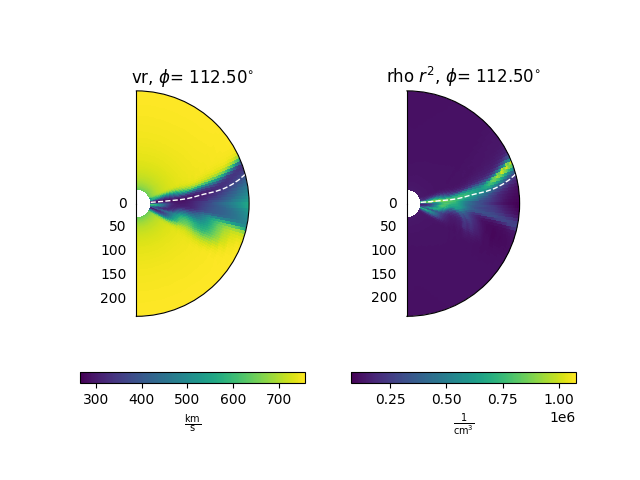# Plotting constant longitude slices

This example shows how to plot slices of constant longitude from a MAS model output.

from psipy.model import MASOutput
from psipy.data import sample_data

import matplotlib.pyplot as plt


Next, load a set of MAS output files. You will need to change this line to point to a folder with MAS files in them.

mas_path = sample_data.mas_helio()
model = MASOutput(mas_path)


Each MAS model contains a number of variables. The variable names can be accessed using the .variables attribute.

print(model.variables)


Out:

['br', 'rho', 'vr']


Set parameters for plotting. The first line will give us a horizontal errorbar underneath the plots. The second line is the index to select for the longitude slice.

cbar_kwargs = {'orientation': 'horizontal'}
phi_idx = 40


Plot the slices

Note that for density (rho) and pressure (p) we first normalise the data relative to a power law decrease, to make it easer to see spatial variations.

fig = plt.figure()
axs = [plt.subplot(1, 2, i + 1, projection='polar') for i in range(2)]

ax = axs
model['vr'].plot_phi_cut(phi_idx, ax=ax, cbar_kwargs=cbar_kwargs)

ax = axs
rho = model['rho']
rho_r2.plot_phi_cut(phi_idx, ax=ax, cbar_kwargs=cbar_kwargs)

# Add a contour of br = 0 (the heliopsheric current sheet) to all the axes
for ax in axs:
model['br'].contour_phi_cut(phi_idx, levels=, ax=ax,
colors='white', linestyles='--', linewidths=1)

plt.show()Total running time of the script: ( 0 minutes 0.427 seconds)

Gallery generated by Sphinx-Gallery